xMolar concentrationEncyclopedia
In chemistry, the molar concentration,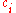is defined as the amount
Amount of substance
Amount of substance is a standards-defined quantity that measures the size of an ensemble of elementary entities, such as atoms, molecules, electrons, and other particles. It is sometimes referred to as chemical amount. The International System of Units defines the amount of substance to be...

of a constituent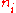divided by the volume
Volume
Volume is the quantity of three-dimensional space enclosed by some closed boundary, for example, the space that a substance or shape occupies or contains....

of the mixture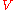: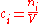It is also called molarity, amount-of-substance concentration, amount concentration, substance concentration, or simply concentration. The volumein the definition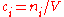refers to the volume of the solution, not the volume of the solvent. One litre of a solution usually contains either slightly more or slightly less than 1 litre of solvent because the process of dissolution causes volume of liquid to increase or decrease.

## Units

The SI-unit
International System of Units
The International System of Units is the modern form of the metric system and is generally a system of units of measurement devised around seven base units and the convenience of the number ten. The older metric system included several groups of units...

is mol/m3. However, more commonly the unit mol/L
Litre
pic|200px|right|thumb|One litre is equivalent to this cubeEach side is 10 cm1 litre water = 1 kilogram water The litre is a metric system unit of volume equal to 1 cubic decimetre , to 1,000 cubic centimetres , and to 1/1,000 cubic metre...

is used.
A solution of concentration 1 mol/L is also denoted as "1 molar" (1 M).
1 mol/L = 1 mol/dm
Decimetre
A decimetre is a unit of length in the metric system, equal to one tenth of a metre, the SI base unit of length. In simple words there are 10 cm in a decimetre....

3 = 1 mol dm−3 = 1 M = 1000 mol/m3.

An SI prefix
SI prefix
The International System of Units specifies a set of unit prefixes known as SI prefixes or metric prefixes. An SI prefix is a name that precedes a basic unit of measure to indicate a decadic multiple or fraction of the unit. Each prefix has a unique symbol that is prepended to the unit symbol...

is often used to denote concentrations. Commonly used units are listed in the table below:
Name Abbreviation Concentration Concentration (SI-unit)
millimolar mM 10−3 mol/dm3 100 mol/m3
micromolar μM 10−6 mol/dm3 10−3 mol/m3
nanomolar nM 10−9 mol/dm3 10−6 mol/m3
picomolar pM 10−12 mol/dm3 10−9 mol/m3
femtomolar fM 10−15 mol/dm3 10−12 mol/m3
attomolar aM 10−18 mol/dm3 10−15 mol/m3
zeptomolar zM 10−21 mol/dm3 10−18 mol/m3
yoctomolar yM 10−24 mol/dm3
(1 molecule per 1.6 L)
10−21 mol/m3

### Number concentration

The conversion to number concentration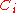is given by: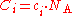where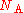is the Avogadro constant, approximately 6.022 mol
Mole (unit)
The mole is a unit of measurement used in chemistry to express amounts of a chemical substance, defined as an amount of a substance that contains as many elementary entities as there are atoms in 12 grams of pure carbon-12 , the isotope of carbon with atomic weight 12. This corresponds to a value...

−1.

### Mass concentration

The conversion to mass concentration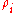is given by: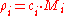where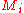is the molar mass
Molar mass
Molar mass, symbol M, is a physical property of a given substance , namely its mass per amount of substance. The base SI unit for mass is the kilogram and that for amount of substance is the mole. Thus, the derived unit for molar mass is kg/mol...

of constituent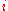.

### Mole fraction

The conversion to mole fraction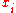is given by: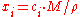where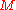is the average molar mass of the solution and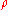is the density
Density
The mass density or density of a material is defined as its mass per unit volume. The symbol most often used for density is ρ . In some cases , density is also defined as its weight per unit volume; although, this quantity is more properly called specific weight...

of the solution.

### Mass fraction

The conversion to mass fraction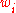is given by: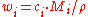### Molality

The conversion to molality (for binary mixtures) is: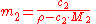where the solute is assigned the subscript 2.

For solutions with more than one solute, the conversion is: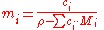### Dependence on volume

Molar concentration depends on the variation of the volume of the solution due mainly to thermal expansion.

## Examples

Example 1: Consider 11.6 g of NaCl dissolved in 100 g of water. The final mass concentration(NaCl) will be:(NaCl) = 11.6 g / (11.6 g + 100 g) = 0.104 g/g = 10.4 %

The density of such a solution is 1.07 g/mL, thus its volume will be:= (11.6 g + 100 g) / (1.07 g/mL) = 104.3 mL

The molar concentration of NaCl in the solution is therefore:(NaCl) = 11.6 g / (58 g/mol * 104.3 mL) = 0.00192 mol/mL = 1.92 mol/L

Here, 58 g/mol is the molar mass of NaCl.

Example 2: Another typical task in chemistry is the preparation of 100 mL (= 0.1 L) of a 2 mol/L solution of NaCl in water. The mass of salt needed is:(NaCl) = 2 mol/L * 0.1 L * 58 g/mol = 11.6 g

To create the solution, 11.6 g NaCl are placed in a volumetric flask
A volumetric flask is a piece of laboratory glassware, a type of laboratory flask, used in analytical chemistry for the preparation of solutions. It is made of glass or plastic and consists of a flat bottomed bulb with a long neck, usually fitted with a stopper. The stopper is normally made in a...

, dissolved in some water, then followed by the addition of more water until the total volume reaches 100 mL.

Example 3: The density of water
Water
Water is a chemical substance with the chemical formula H2O. A water molecule contains one oxygen and two hydrogen atoms connected by covalent bonds. Water is a liquid at ambient conditions, but it often co-exists on Earth with its solid state, ice, and gaseous state . Water also exists in a...

is approximately 1000 g/L and its molar mass is 18.02 g/mol. Therefore, the molar concentration of water is:(H2O) = 1000 g/L / (18.02 g/mol) = 55.5 mol/L

Likewise, the concentration of solid hydrogen
Solid hydrogen
Solid hydrogen is the solid state of the element hydrogen, achieved by decreasing the temperature below hydrogen's melting point of 14.01 K . It was collected for the first time by James Dewar in 1899 and published with the title "Sur la solidification de l'hydrogène" in the Annales de Chimie et...

(molar mass = 2.02 g/mol) is:(H2) = 88 g/L / (2.02 g/mol) = 43.7 mol/L

The concentration of pure osmium tetroxide (molar mass = 254.23 g/mol) is:(OsO4) = 5.1 kg/L / (254.23 g/mol) = 20.1 mol/L.

Example 4: Proteins in bacteria
Bacteria
Bacteria are a large domain of prokaryotic microorganisms. Typically a few micrometres in length, bacteria have a wide range of shapes, ranging from spheres to rods and spirals...

, such as E. coli, usually occur at about 60 copies, and the volume of a bacterium is about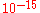L. Thus, the number concentration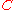is:= 60 / (10−15 L)= 6 L−1

The molar concentration is: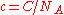= 6 L−1 / (6 mol−1) = 10−7 mol/L = 100 nmol/L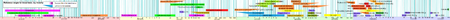If the concentration refers to original chemical formula in solution, the molar concentration is sometimes called formal concentration. For example, if a sodium carbonate solution has a formal concentration of(Na2CO3) = 1 mol/L, the molar concentrations are(Na+) = 2 mol/L and(CO32-) = 1 mol/L because the salt dissociates into these ions.